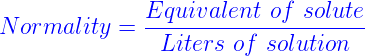# Normality Formula

Normality indicates the concentration of the solution. Normality definition is the weight of solute dissolved in a liter of solution. Normality of a solution can be calculated when atomic mass and volume are known. If the composition of solute and solvent are same, the solution is saturated.

Normality formula is given by,Multiplying molarity can consider favorable normality calculation by the number of equivalents.
Normality (N) = Molarity (M) x number of equivalents
Example 1

Determine the normality of 0.1381 mol of NaOH, which is dissolved in 300 mL.
Solution- Equivalent solute = 0.1381mol x 1 eq/mol

Solution = 300mL = 0.300L

Normality = 0.1381 x 1 eq/mol 0.300 L

Therefore Normality = 0.460 N
Example 2- Determine the normality of 0.248mol of H2SO4, which is dissolved in 250mL of solution.

Solution

Equivalent solute = 0.248mol x 2eq/mol

Solution = 250 mL = 0.250L

Normality = 0.248mol x 2 eq /mol 0.250L

Therefore Normality = 1.984 N
To solve more problems on Normality formula and practice more questions, please visit Byju’s.com

#### Practise This Question

A coil of 100 turns carries a current of 5mA and creates a magnetic flux of 105 Weber. The inductance is